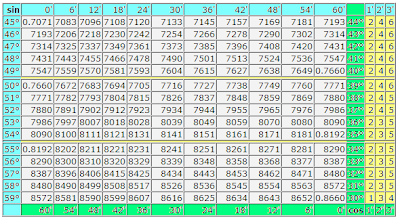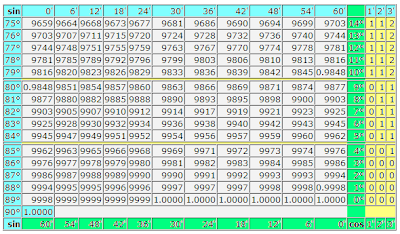## 1.10.2012

### Trigonometric table of sines and cosines

The trigonometric table of values of sines and cosines within one minute is counted on blondes. For comfort of the use for sines and cosines distinguished this table of value of corners by different colors. For sines the blue color of cells is accepted with degrees and by minutes. For cosines the green background of cells is accepted. A yellow background is distinguish the values of minutes that if necessary is added or subtracted from tabular values.Usually it is not accepted so in detail to give a navigation on a trigonometric table. Firstly, a table is counted on experience users by mathematics. Secondly, publishers from old times produce these tables in a blackly white variant and in every way save printer's ink on a navigation on a trigonometric table.

I hope, such registration will not give to lose way you even in the middle of this table and you will not entangle sines with cosines at the search of their values. By the way, the trigonometric table of sines and cosines presents the values of these trigonometric functions for corners from 0 to 90 degrees. For other values of corners can use a trigonometric circle as a crib.

If you need more exact calculation of values of trigonometric functions, then can take advantage of calculator. How to use the table of Брадиса, we will understand next time. Specially for those, whoever knows.